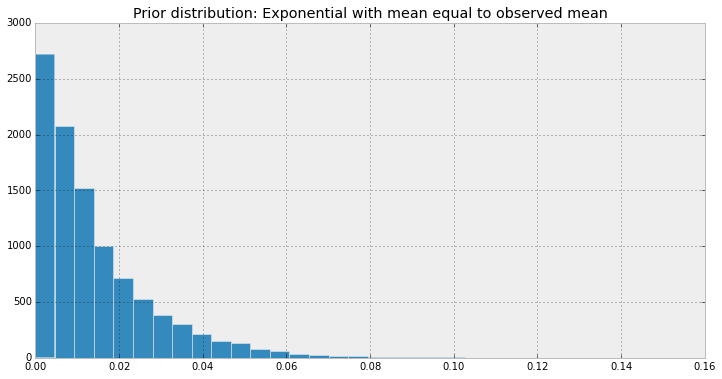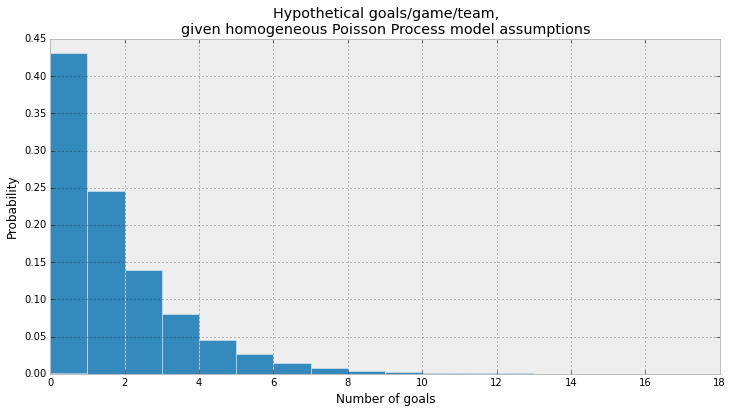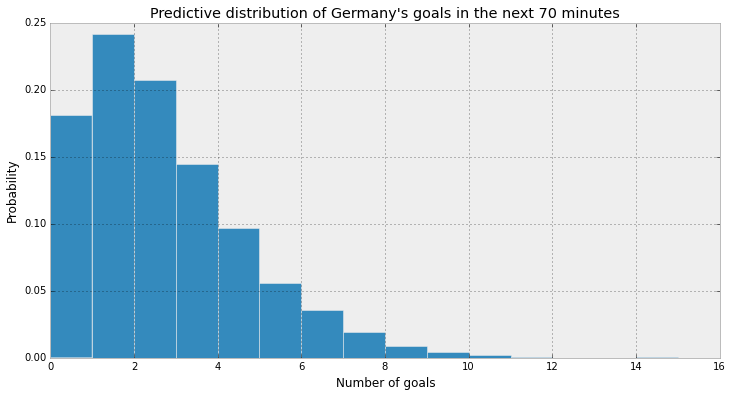## The World Cup Problem: Germany v. Brazil¶

PyMC solution to Allen D.s soccer problem.

We'll use an exponential prior, instead of a Uniform prior, but also with mean equal to the observed sample mean goals per team per game.

In :
import pymc as pm
%pylab inline
figsize(12,6)

Populating the interactive namespace from numpy and matplotlib

In :
avg_goals_per_team = 1.34
duration_of_game = 93.

In :
#prior
lambda_ = pm.Exponential('lambda_', duration_of_game/avg_goals_per_team)
sample = np.array([lambda_.random() for i in range(10000)])
hist(sample, bins=30);
plt.title('Prior distribution: Exponential with mean equal to observed mean');In :
sample_points_per_team = np.random.poisson(duration_of_game*sample)
hist(sample_points_per_team, bins=sample_points_per_team.max(), normed=True);
plt.title('Hypothetical goals/game/team,\ngiven homogeneous Poisson Process model assumptions')
plt.ylabel('Probability')
plt.xlabel('Number of goals');

print sample_points_per_team.mean()

1.3133In :
duration_between_goals = [11, 12]

obs = pm.Exponential('obs', lambda_, observed=True, value=duration_between_goals)

prediction = pm.Poisson('pred', (duration_of_game-23)*lambda_)

mcmc = pm.MCMC([lambda_, obs, prediction])
mcmc.sample(15000,5000)

 [-----------------100%-----------------] 15000 of 15000 complete in 2.0 sec
In :
prediction_trace = mcmc.trace('pred')[:]
hist(prediction_trace,bins=max(prediction_trace), normed=True);
plt.title("Predictive distribution of Germany's goals in the next 70 minutes")
plt.ylabel('Probability')
plt.xlabel('Number of goals');In :
(prediction_trace >= 5).mean()

Out:
0.128

We get more than twice the probability that Germany scores 5 or more goals. Why such a large difference? Since we have very few data points (only two), our prior still has lots of influence on the posterior, so our results are very depenedent on prior chosen - and Allen and I choose different priors (uniform vs. exponential).

In [ ]: